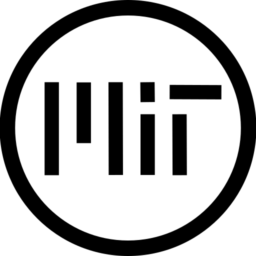# Rounded corners

``````shader_type canvas_item;
// Shader to round corners of a canvas. The 'radius_scale' is multiplied by
// minimum(width, height) to calculate the radius of the corners.
//
// Instructions:
// 1) The node that uses this shader must have the next signal connection (
// using node size is better than its texture size when (width >> height) or
// (height >> width) to get better corners). Signal 'item_rect_changed', code:
//
// Known issues:
// 1) If used on 'TextureRect' with 'strecth_mode = keep_aspect_covered',
// texture borders might be not visible, since they are outside the node
// rectangle (although they are rounded).
// 2) It is recommended to reload the scene if you changed the node properties
// and you get weird results in the Editor. It seems that they are not updated
// correctly.

uniform float radius_scale: hint_range(0.0, 1.0, 0.1) = 0.1;
uniform bool rounded_top_left_corner = true;
uniform bool rounded_top_right_corner = true;
uniform bool rounded_bottom_left_corner = true;
uniform bool rounded_bottom_right_corner = true;
uniform float width = 1.0;
uniform float height = 1.0;

void fragment() {
vec4 image = texture(TEXTURE, UV);
vec2 pos = vec2(UV.x*width, UV.y*height);
float dist;
// Top left corner
if (rounded_top_left_corner) {
image.a = 0.0;
}
// debugging only
//		if (dist < radius){image.r = 1.0;}
}
// Top right corner
if (rounded_top_right_corner) {
image.a = 0.0;
}
// debugging only
//		if (dist < radius){image.r = 1.0;}
}
// Bottom left corner
if (rounded_bottom_left_corner) {
image.a = 0.0;
}
// debugging only
//		if (dist < radius){image.r = 1.0;}
}
// Bottom right corner
if (rounded_bottom_right_corner) {
image.a = 0.0;
}
// debugging only
//		if (dist < radius){image.r = 1.0;}
}
COLOR = image;
}``````
###### Tags
corner, RoundedThe shader code and all code snippets in this post are under MIT license and can be used freely. Images and videos, and assets depicted in those, do not fall under this license. For more info, see our License terms.
Subscribe
Notify of